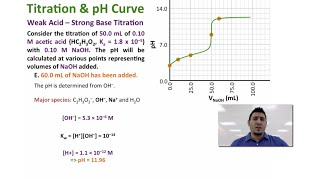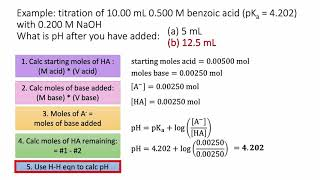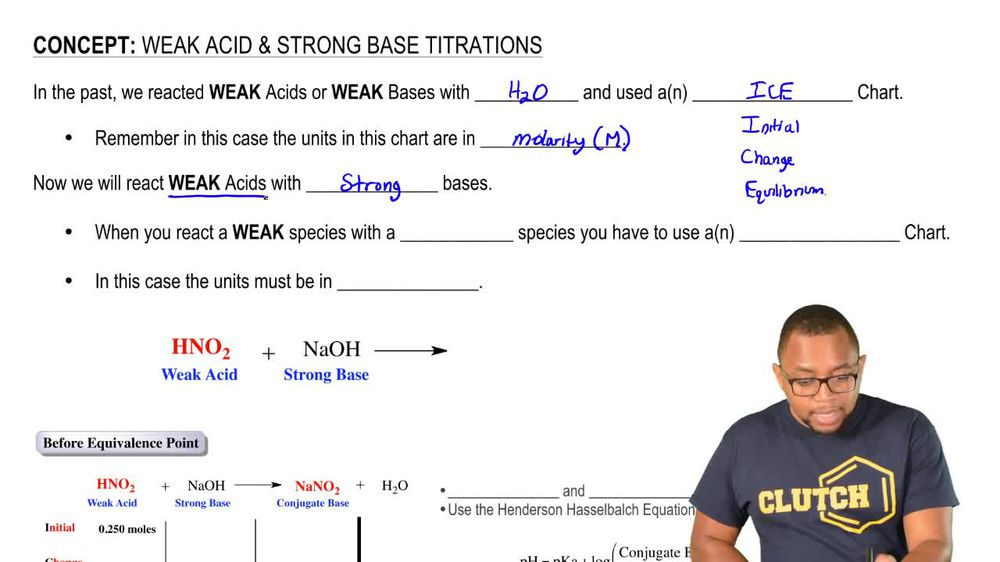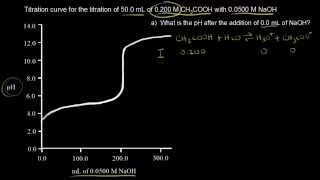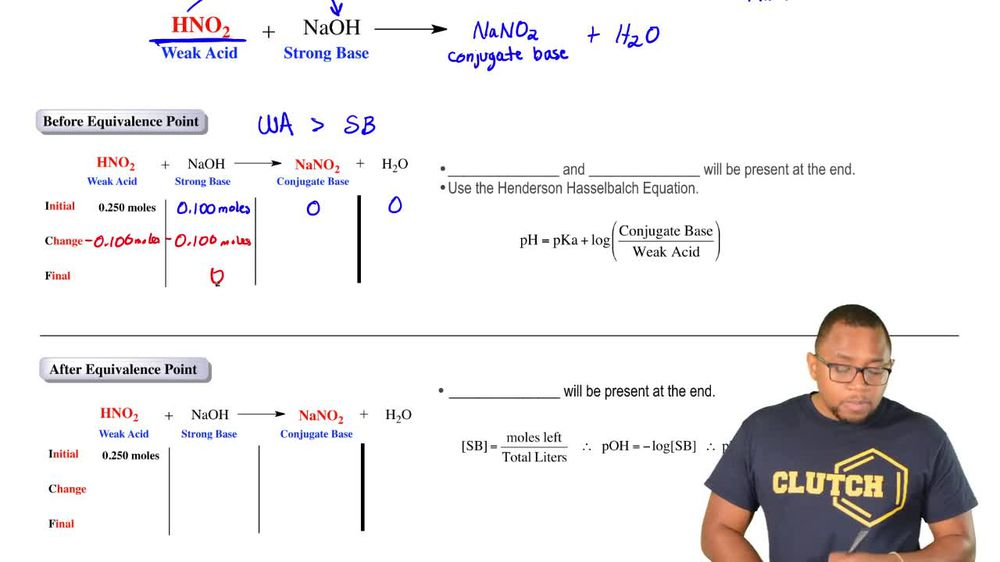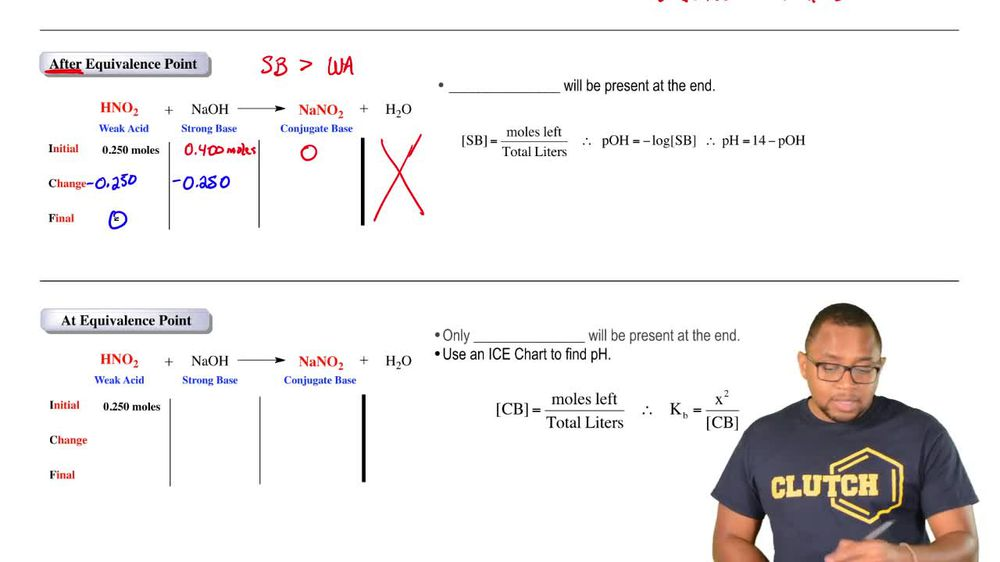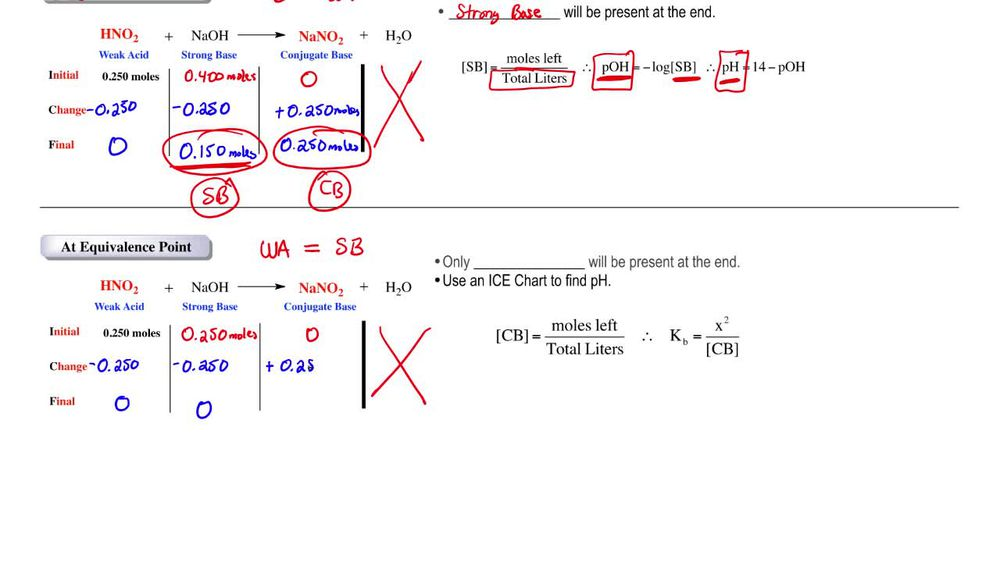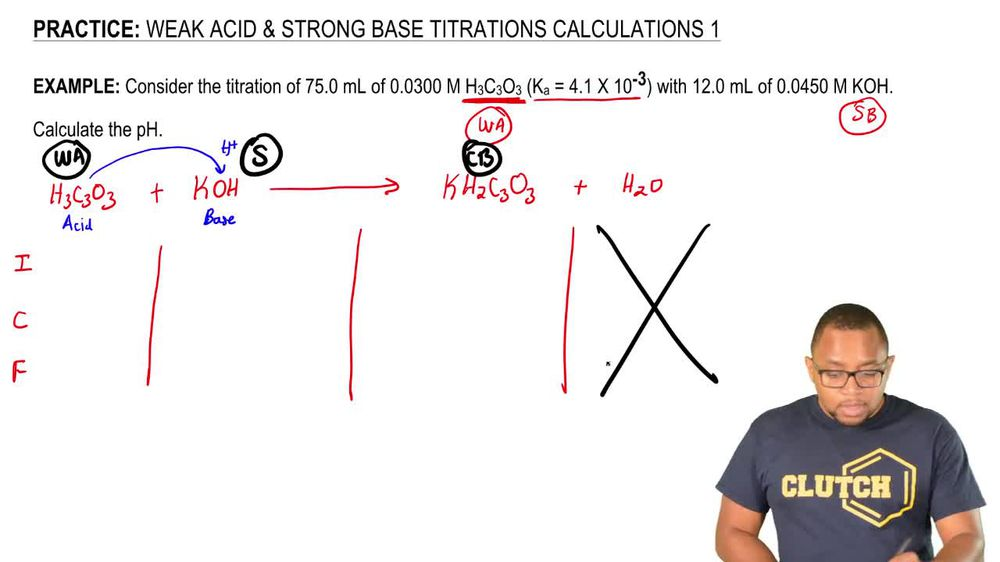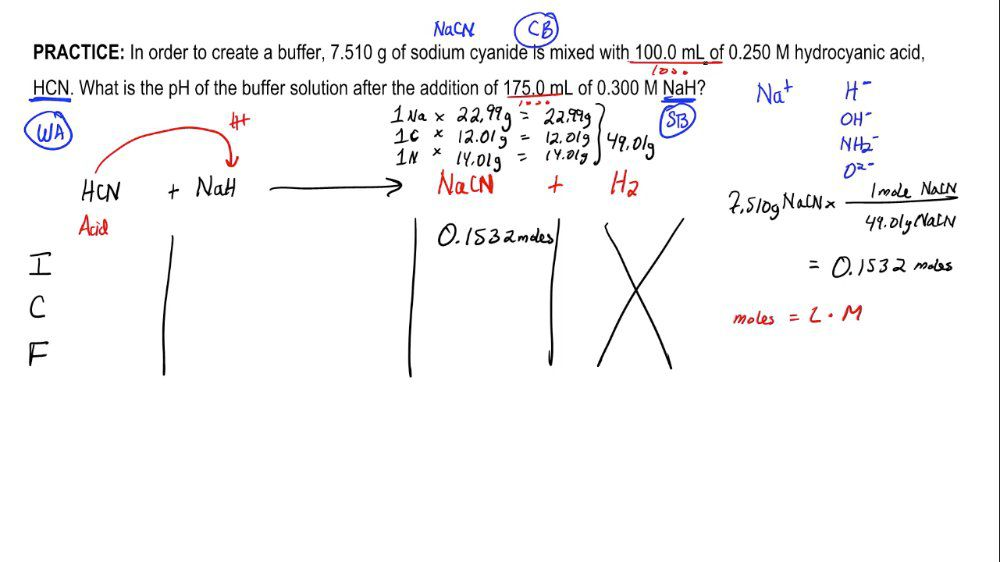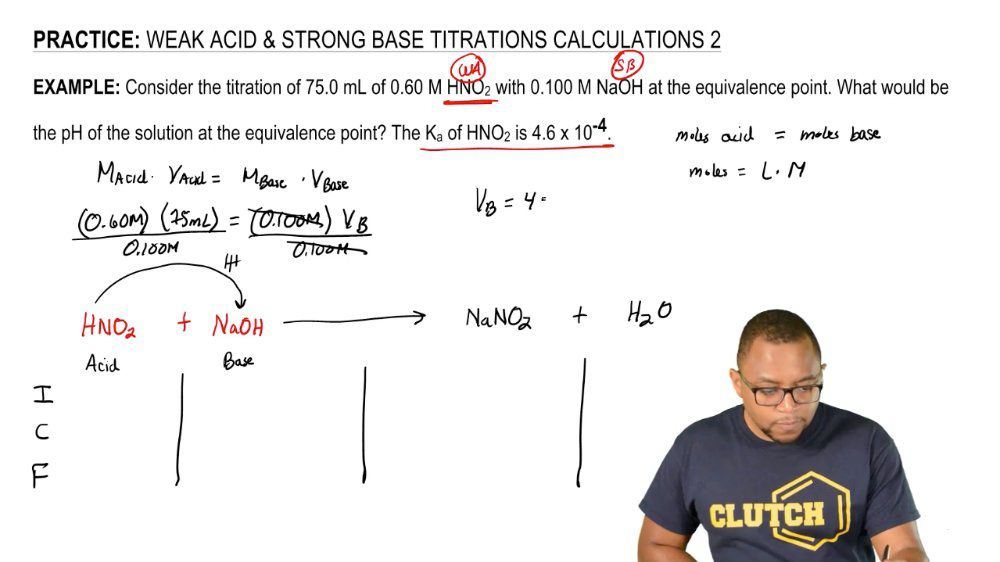Start typing, then use the up and down arrows to select an option from the list.
1. 18. Aqueous Equilibrium2. Weak Acid Strong Base Titrations# Weak Acid-Strong Base Reactions Concept 4

by Jules Bruno
176 views
2
0
in this third situation, we're now at the equivalence point At the equivalence point are weak species which is our week. Answered in this case is equal in terms of amount toe are strong species which is our strong base. So here would be the same exact moles as we have for the weak acid. And again we look at the react inside the smaller moles of track from the larger moles. But here they both have the same exact mall, so they completely destroy each other again. Remember conservation of mass. That means we're adding 2.250 moles to the product side. So at the end, all we have left is our conjugated base. So on Lee conjugate base will be remaining in terms of this situation. Conjugate base is equal to a week base. Remember, for weak acids and weak bases. In order to determine ph, we have to use a nice chart. So what we would do is we take the most left of our conjugate base and divided by the total leaders used in the question to find our polarity. We then set up an ice chart in order to determine our equilibrium expression because it's a week base, we'd utilize K B. Now, as we delve deeper into the situation of a weak, acid, strong based titrate Asian, we'll see examples that fit into these three situations. Right now, we're just laying down the groundwork for the three situations that arise, um, involving the Thai tray shin of a weak acid in a strong base. So just keep in mind what happens before the equivalents point after the equivalents point and at the equivalents point when these types of questions arise later on within our lesson.